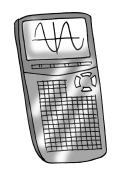### Home > APCALC > Chapter 4 > Lesson 4.4.3 > Problem4-164

4-164.

Set up an integral and compute the area of the region bounded by the graphs of the given functions. You may use a graphing calculator.1. The area between $y=\sin\left(x\right)$ and $y = \left(x – 1\right)^{4} – 1$

$\text{Area between curves }=\int_{a}^{b}\text{(upper fuction) - (lower function)}dx$

Find the bounds by computing the $x$-values of the points of intersection between the two functions.
$\sin x=\left(x−1\right)^4−1$
$a = 0, b = 2.163$ (using a calculator)

Determine which is the upper function (the one with higher $y$-values) and which is the lower function (the one with lower $y$-values). If you are using a calculator: look at the graph. If not: Evaluate a point in between the bounds to see which function has the higher $y$-values.

2. The area between $y = x\left(x – 3\right)$ and $y=\sqrt{x}$

Refer to hints in part (a).